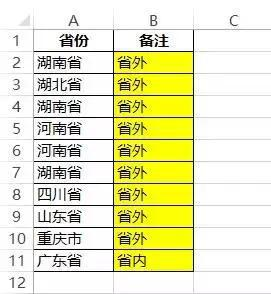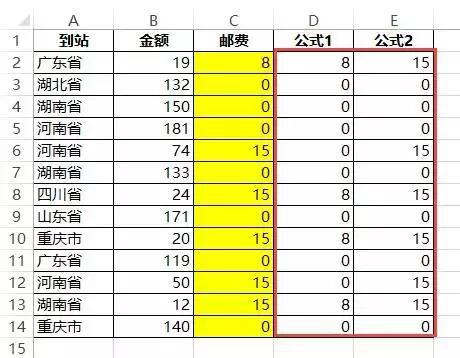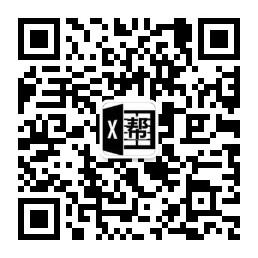# 别怕！人人都可以学好Excel函数与公式 IF函数案例图文讲解

2018年2月20日00:19:57 评论 1,121 views

1、对省份进行判断，广东省的就属于省内，其他属于省外。

=IF(A2="广东省","省内","省外")IF函数语法：

=IF(条件,满足的情况下返回值2,不满足的情况下返回值3)

A2="广东省"就是条件，如果单元格是广东省就返回第2参数也就是省内，否则就返回第3参数省外。

=IF(A2="广东省","省内","")

""就是代表空白，什么都不显示的意思。假设让省外的显示空白，这样就会变得更加清晰，一目了然。2、对省份进行判断，广东省和四川省有熟人，其他省份没有。

=IF(OR(A2="广东省",A2="四川省"),"有熟人","")

OR函数语法：

=OR(条件1,条件2,条件n)

OR函数也可以用+取代。

=IF((A2="广东省")+(A2="四川省"),"有熟人","")

AND函数就是需要满足所有条件才成立。举个最简单的例子，我每天早上发布文章，你每天早上看文章后留言，只有当这两个条件同时满足，才算读者与卢子有了互动，假设任何一方没做到，就不叫互动。

=IF(AND(A1="卢子发文章",B1="读者留言"),"互动","")

AND函数也可以用四则运算的*代替。

=IF((A1="卢子发文章")*(B1="读者留言"),"互动","")

3、计算每一笔快递的邮费，广东省内消费满39元包邮，未满39元邮费8元；其他省份消费满79元包邮，未满79元邮费15元。

=IF(A2="广东省",IF(B2>=39,0,8),IF(B2>=79,0,15))=IF(A2="广东省",公式1,公式2)

=IF(B2>=39,0,8)

=IF(B2>=79,0,15)=IF(A2="广东省",D2,E2)

=IF(A2="广东省",IF(B2>=39,0,8),IF(B2>=79,0,15))

##### 历史上的今天

20
• 郑广学老师微信号
• EXCEL880B
•• 我的微信公众号
• EXCEL880
•